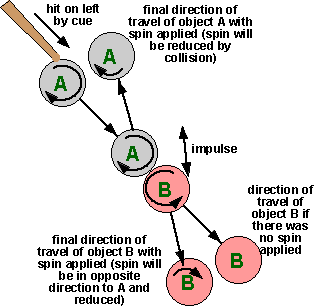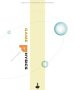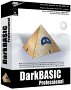# Table Top Physics - Snooker/pool - left side

Ball A is sent toward ball B, initially A has clockwise spin and B has no spin, they hit head-on. Due to the rotation and friction, the impulse is at a different angle to the approach velocity and to surface normal. This impulse tends to reduce the angular speed of A and to cause B to rotate in the opposite direction. If we assume that the initial angular velocity is w, if we assume that the contact is perfect and all the angular velocity is transferred to ball B, and B is rotating in the opposite direction. So the angular momentum of A added to the angular momentum of B will change from +Iw to -Iw.Since angular momentum of a closed system is conserved and we have a change of angular momentum of 2*I*w, where does this angular momentum go?

I think the answer is that we must measure the total angular velocity of the whole system, so let us take the CM of A at the point of collision to measure the total angular velocity. Since A is travelling directly to, and directly from, this point we only need to take into account its rotation. The velocity of Bs CM is offset, so we need to include this.so initial angular velicity = final angular velocity

Iw = -Iw + m v offset

2 I w = m v offset

but for a sphere I = m * 2/5 r2

therefore for angular momentum to be conserved:

offset = 2*I*w / m*v

offset = 2*m * (2/5) r2 *w / m*v

offset = (4/5) r2 *w / v

where:

• r = radius of ball
• w = angular velocity of ball
• v = final velocity of ball B

So we can calulate the offset, and from that we can calculate the angle that ball B is deflected.

Can anyone check these calculations and finish it off?

Where I can, I have put links to Amazon for books that are relevant to the subject, click on the appropriate country flag to get more details of the book or to buy it from them.Game Physics - This book has some useful stuff, its more of a textbook, not a step by step guide (although it does have a disc with a lot of C++ code). About the first third of the book is a physics textbook with theoretical exercises, the middle bit covers physics engine topics, and the last third of the book covers mathematical topics. I think I would use this book as a reference book to lookup the theory behind something I might be working on rather than a book to work through in order.

Commercial Software Shop

Where I can, I have put links to Amazon for commercial software, not directly related to the software project, but related to the subject being discussed, click on the appropriate country flag to get more details of the software or to buy it from them.Dark Basic Professional Edition - It is better to get this professional editionThis is a version of basic designed for building games, for example to rotate a cube you might do the following: make object cube 1,100 for x=1 to 360 rotate object 1,x,x,0 next x

This site may have errors. Don't use for critical systems.